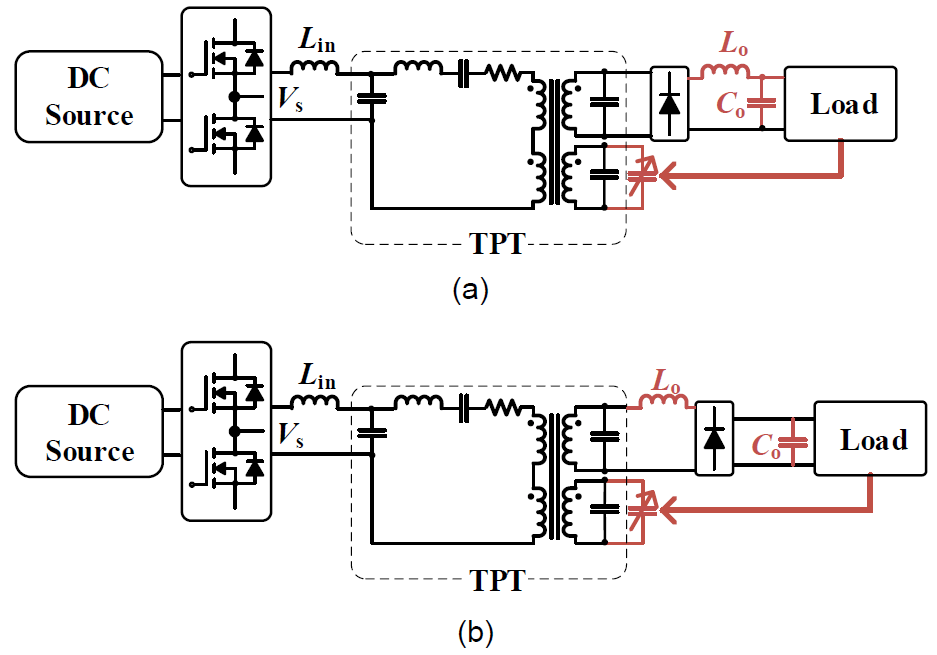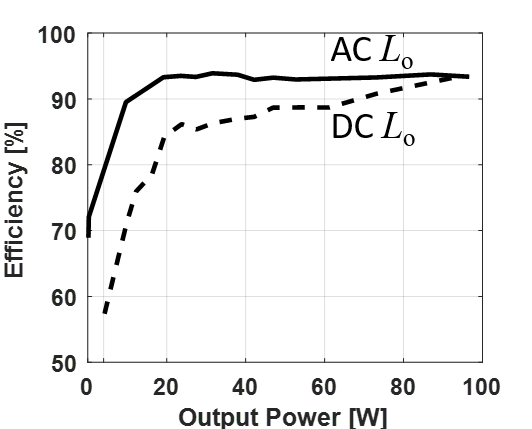LIBRARY

# Analysis and Comparison of DC and AC Output Inductors in Tunable Piezoelectric Transformer Based DC/DC ConvertersFig. 1. TPT-based dc-dc converter with (a) dc output inductor and (b) ac output inductor.
Different from traditional magnetic transformers, which transform the electrical energy via the magnetic flux in the core, piezoelectric transformers (PTs) transform the electrical energy via the mechanical vibration of the piezoelectric material. The tunable piezoelectric transformer (TPT) is a recently developed piezoelectric transformer with three ports: input, output and control ports. It can be treated as the integration of a resonant tank and a transformer, where the series-resonant capacitance can be tuned by the control port. Fig. 1 shows two ways to build a TPT-based dc-dc converter. The only difference is the output inductor. The output inductor in Fig. 1(a) is denoted by dc output inductor since it has a non-zero average current. The output inductor in Fig. 1(b) is denoted by ac output inductor because of its zero average current.
There are two benefits of the ac output inductor. The first is that the ac output inductor helps improve efficiency. The ac output inductor compensates the circulating energy caused by the output capacitor of the TPT. Therefore, theoretically the efficiency can be improved. Secondly, with the help of the ac output inductor, the converter has better regulation ability and requires less from the control. It can be found that, with ac output inductor, the power stage becomes the series composition of an LC tank, a series resonant tank and a CL tank. If the LC tank and the CL tank have the same resonant frequency as the switching frequency, then the input-to-output gain of the power stage will be load independent.
Fig. 2 shows the measured efficiency curves of the TPT-based converters with a dc output inductor and an ac output inductor. It can be verified that the one with ac output inductor has wider regulation range and better efficiency, especially light load efficiency.Fig. 2. Measured converter efficiency.